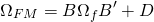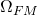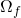# Factor risk model

A factor risk model is a method used by investors to estimate the riskiness and relationship between securities. In particular, a factor risk model allows investors to construct the covariance matrix of the assets in the portfolio. Estimating the covariance matrix is notoriously difficult because we need considerable amounts of data to estimate all the covariance terms.

On this page, we discuss the different types of factor risk models and explain the advantages and disadvantages of the different factor risk model approaches.

## Factor risk model definition

The factor risk model is defined as followswhereis the covariance matrix we are interested in, B is the matrix with the exposures of the securities to the factors,is the covariance matrix of the factors, and D is the diagonal matrix containing the asset specific risks.

## Factor risk model estimation

Thus, to create a factor risk model, we need to do the following. First, we need to define the relevant factors. These factors can be macroeconomic factors, in which case the model is macroeconomic factor risk model. Alternatively, the model can use stock fundamentals, in which case we have a fundamental factor risk model. A good example of a fundamental risk model is the Barra risk model owned by MSCI. Third, we can estimate the factors from the data first using a dimensionality reduction method such as Principal Component Analysis (PCA). The principal components are then the factors in our model. This is a statistical factor risk model.

Once we have the factors, the next step is to calculate the exposure of the stocks to the factors. To do that, we can make use of linear regression. These exposure are then our B. Since we already have the factors using one of three different approaches, we can also calculate the covariance matrix of the factors. Third, we still have D, which are the asset-specific risk. To calculate these we can calculate the total risk of each stock (its standard deviation) and subtract the first part of the above equation. The difference can then be used as the asset-specific risk.

## Summary

Investors can use different factor risk models for different reasons. The advantage of fundamental factor risk model is that the results are easy to interpret because the factors have a clear economic meaning. This explains why most investors use these models. The most famous fundamental risk model is the BARRA risk model. Other providers are Axioma and Northfield.

The statistical risk factor model is easier to implement, since that only requires return data. The factors are built from the data. Some managers do not like the use of statistical factors because they cannot be interpreted that easily. Macroeconomic factor models are typically not used, because it is not easy to find all the appropriate factors that drive security returns.